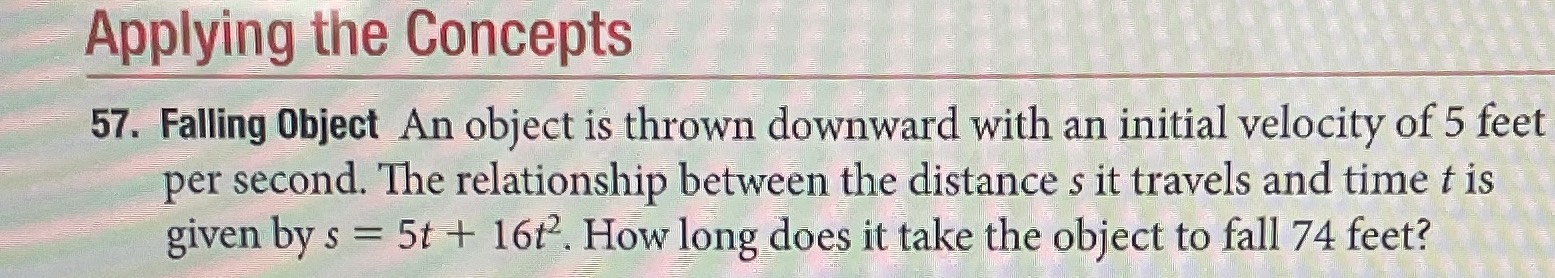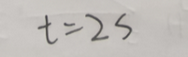### ¿Todavía tienes preguntas de matemáticas?

Pregunte a nuestros tutores expertos
Algebra
PreguntaFalling object An object is thrown downward with an initial velocity of $$5$$ feet per second. The relationship between the distance $$s$$ it travels and time $$t$$ is given by $$s = 5 t + 16 t ^ { 2 }$$ . How long does it take the object to fall $$74$$ feet?